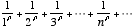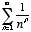index: click on a letter A B C D E F G H I J K L M N O P Q R S T U V W X Y Z A to Z index index: subject areas numbers & symbols sets, logic, proofs geometry algebra trigonometry advanced algebra & pre-calculus calculus advanced topics probability & statistics real world applications multimedia entrieswww.mathwords.com about mathwords website feedback

 p-series A series of the formor, where p > 0. Often employed when using the comparison test and the limit comparison test. Note: The harmonic series is a p-series with p =1.See also